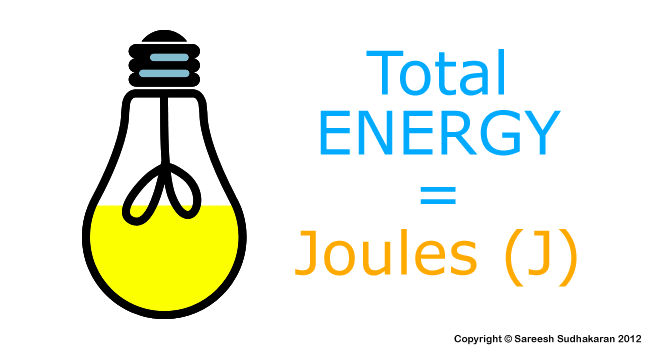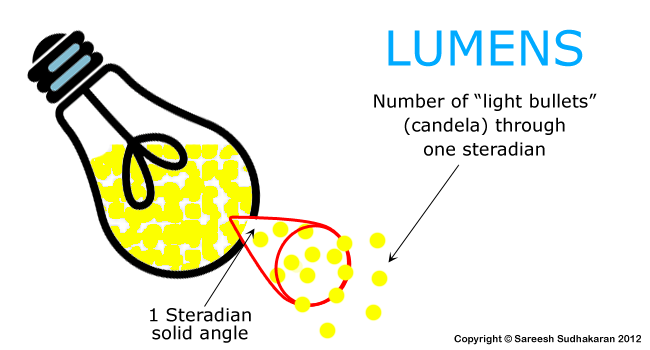# Notes by Dr. Optoglass: The Units of Photometry – Part I

Topics Covered:

• Joules and Watts
• Lumens and Candelas
• Lumens per Watt
• Counting Photons

A beautiful thing never gives so much pain as does failing to hear and see it – Michelangelo

Let’s start with some radiometry units:Imagine a light bulb has having a total volume (energy) of light that it can pour out during its lifetime. This total ‘volume’ is called the Radiant Energy, and is measure in Joules.

If you’ve looked at your electricity bills, you’ll realize that power companies charge in KWh – which is a unit of energy. How many watts have you used, over how much time, gives the total energy. Similarly, it is convenient to rate ‘energy-giving’ objects in terms of their Power (energy / time).

This, for a source of light, is officially called Radiant Flux and is measured in Watts. This is the most traditionally used rating for light bulbs. It was good enough in the earlier days, since most bulbs were incandescent and if you told someone: “This bulb is 100 Watts” it was pretty much understood how much light can be expected from such a bulb.

However, in today’s world, there are many kinds of light sources – incandescent, HMI, fluorescent, LED, laser, etc. Many of these lights are still sold with Wattage ratings, but they don’t mean the same thing anymore.

What we need is a way to equate these varying sources into visible light, so we can judge correctly.

The simplest way to count light is by counting the number of photons.

Photons

Photons are wherever EMR is – visible or invisible. The energy in one photon depends on its frequency as per the formula:

E = h?

where h is the Planck’s constant = 6.626 x 10-34 Joule seconds (Js)
? is the frequency of light in per seconds (1/s)

The frequency of light is related to its wavelength by the formula:

? = c / ?

where

• c is the speed of light = 3 x 108 m/s
• ? is the wavelength in m

From this, we derive the formula for energy as:

E = hc/?

Since we are only concerned with photons in the visible spectrum, our values of ? lie in the range 390 x 10-9 m to 750 x 10-9 m. Therefore, our photon can have an energy between 2.5 to 5 x 10-19 J.

If a light bulb is rated at 100W, then its energy per second is 100 J. Therefore the number of photons that the light bulb emits per second is 2 to 4 x 1018. Whoa! That’s a very large number. To understand the magnitude of this figure, just realize that even if we miscounted the number of photons by a few trillions, it would be a negligible error! And this value is just for one second!

How do we bring it down to a more manageable level? Enter photometric units:

Luminous Flux (Lumens or lm)

Look on the back of a good light bulb. You should see its power rating in Watts. You should also see a number in lumens (lm). Let’s say your bulb is CFL – 5W at 235 lm.

Luminous flux is the photometric counterpart of Watts. If watts is a measure or total power, then lumens is a measure of perceived power of light.So, if we had a handle on “light bullets” (or Candela – we’ll come to that soon) through a “funnel” of solid angle of one steradian (solid angles are used in 3D space, as opposed to radians in 2D), we know the luminous power (luminous flux) of that source.

Power is dependent on time, brightness is not. Luminous flux eliminates the need to consider time, and directly takes us to a unit that deals with visible light.

The lumens mentioned on a light bulb is the total luminous flux of that bulb. The next step is to find the relation between Watts and Lumens.

Luminous Efficacy

If you continue further on the ratings on the light bulb, you’ll also see a value next to the unit lm/W (lumens per Watt). This is called the luminous efficacy. In our example case, the luminous efficacy is 235/5 = 47 lm/W.

What does that tell us? If I had two bulbs:

• 15W CFL bulb rated at 1005 lm or 67 lm/W (LPW), and
• 15W Incandescent bulb rated at 108 lm or 7.2 LPW

Which one is more efficient? The CFL bulb – the higher the LPW the more efficient the bulb. The most efficient LPW values for consumer bulbs are obtained by LED lights.

Candela

Candela is a bullet ray of light – it has a precise definition:

The candela is the luminous intensity, in a given direction, of a source that emits monochromatic radiation of frequency 540×1012 hertz and that has a radiant intensity in that direction of 1/683 watt per steradian.

It is like the SI unit of length, which is meter. If one can produce a light source that is 1 candela (cd), then it can be compared to the test source for calibration. The relationship between candela and lumens is:

Lumens = Candela x 2? (1 – cos(A/2))

where A is the radiation angle of the lamp. For a lamp that emits in 360o, the formula boils down to:

Lumens = Candela x 4? = 12.57 x Candela

Incidentally, one candela is roughly equal to the light produced by one candle – but today it has nothing to do with candles.

So which is it? Which one should you use: Lumens or Candela?

Use both! Candela is a precisely measurable unit in one direction, while lumens depends on candela and the radiation angle. Lumens is more ‘spread out’, if you will. As a very general rule of thumb, candela is used when precise measurements are required, and lumens is used for general sources of light that tends to spread out.

Lumens and Candelas are great to measure light from the source. But brightness has one important factor: Distance. Sometimes it is much better to know how much light is hitting a particular spot, rather than know what the bulb is outputting.

In order to do this, there are two ways: the candela way and the lumens way.

Takeaways:

• Radiant Flux or Power is measured in Watts.
• Counting photons is not practical.
• The lumens mentioned on a light bulb is the total luminous flux of that bulb.
• The Lumens per Watt rating of a bulb tells us its efficiency.
• Candela is a precise measurement of luminous intensity, and is related to lumens.

Links for further study:

Next: The Units of Photometry Part II
Previous: How to Measure Light

## 2 replies on “Notes by Dr. Optoglass: The Units of Photometry – Part I”

1. Marco Chiorri says:

How I can to change 1J(Joule ) to 1cd/m2 ? Very thanks

2. Abdullah Al Fahim says:

Maths!!!!!!!!!!!! :(

Comments are closed.